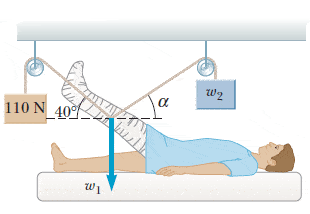# Determining the weight and angle of an object - Help please

riseofphoenix
Determining the weight and angle of an object -- Help please

2. The leg and cast in the figure below weigh 158 N (w1). Determine the weight w2 and the angle α needed so that no force is exerted on the hip joint by the leg plus cast.w2 = ________ N
α = ________ °

OK so I figured out how to do a problem similar to this just a few minutes ago on my own (:D I was so happy), but I have no idea how to solve for this one...
I need help!

## Answers and Replies

Try drawing component forces(sideways and upwards) from each of the lines at the point where they meet.
The sideways ones should cancel each other, and the sum of the upward components should equal the weigh of the leg(so that there is no net force acting on the leg).
The rest is just delicious, delicious trigonometry.

riseofphoenix

Try drawing component forces(sideways and upwards) from each of the lines at the point where they meet.
The sideways ones should cancel each other, and the sum of the upward components should equal the weigh of the leg(so that there is no net force acting on the leg).
The rest is just delicious, delicious trigonometry.

I don't get it though...

You're saying

w3 + w2 = w1???
I tried doing 110 + w2 = 158
w2 = 158 - 110
w2 = 48 N

But that's wrong...

And then I tried doing

w3 = 110 sin 48 = 70
w1 -158 sin 270 = 158

And then I tried doing 158 - 70 = 88 N, which is wrong too...

????

You have to realise that the two forces exerted by the lines on the leg partially cancel each other, so you can't just add them.

In another situation, where both α & β would be equal to 0°, i.e. the lines would pull in the opposite direction, then as long as both weights w2 & w3 were equal, you'd have their forces cancel each other completely.
But then the leg would fall down, because there'd be nothing to counteract it's own weight(w1).

Here, you have a situation where part of the force provided by each of the weights pulls the leg horizontally - and cancels with the other force's horizontal component(otherwise the leg would feel a net force, and the injured guy would cry like a baby). The remaining, vertical components of each of the force add up to counterweigh the leg's downward pull.

What you need to do, is split the two force vectors(that's basically what the lines represent) into their horizontal and vertical components via right angle trigonometry.
If you're not sure what I'm talking about here, browse through this page:
http://zonalandeducation.com/mstm/physics/mechanics/forces/forceComponents/forceComponents.html

Since you know that the leg needs to feel no force at all, you can now write two equations. One for the two horizontal components, that you've just separated, cancelling out each other(i.e. they need to be equal and have opposite direction).
The second one for the two vertical components canceling out the weight of the leg(i.e.their sum must equal the leg's weight).